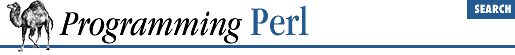#Chapter 3Functions### 3.2.156 sprintf

`sprintf `FORMAT`, `LIST``

This function returns a string formatted by the usual printf conventions. The `FORMAT` string contains text with embedded field specifiers into which the elements of `LIST` are substituted, one per field. Field specifiers are roughly of the form:

`%`m.nx``

where the `m` and `n` are optional sizes whose interpretation depends on the type of field, and `x` is one of:

CodeMeaning
`c`Character
`d`Decimal integer
`e`Exponential format floating-point number
`f`Fixed point format floating-point number
`g`Compact format floating-point number
`ld`Long decimal integer
`lo`Long octal integer
`lu`Long unsigned decimal integer
`lx`Long hexadecimal integer
`o`Octal integer
`s`String
`u`Unsigned decimal integer
`x`Hexadecimal integer
`X`Hexadecimal integer with upper-case letters

The various combinations are fully documented in the manpage for printf(3), but we'll mention that `m` is typically the minimum length of the field (negative for left justified), and `n` is precision for exponential formats and the maximum length for other formats. Padding is typically done with spaces for strings and zeroes for numbers. The `*` character as a length specifier is not supported. But, you can easily get around this by including the length expression directly into `FORMAT`, as in:

```\$width = 20; \$value = sin 1.0;
foreach \$precision (0..(\$width-2)) {
\$output_arr[\$precision] = sprintf "%\${width}.\${precision}f", \$value;
}```